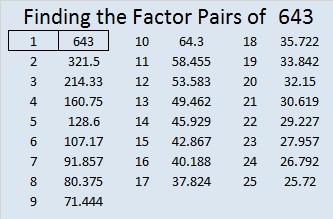# 643 and Level 1

Here’s an interesting prime factorization: 2⁶⋅3⋅643 = 123456. Did you notice its largest prime factor? Thank you OEIS.org for that fun fact about the number 643.Print the puzzles or type the solution on this excel file: 10 Factors 2015-10-12

—————————————————————————————————

• 643 is a prime number. 641 and 643 are twin primes.
• Prime factorization: 643 is prime.
• The exponent of prime number 643 is 1. Adding 1 to that exponent we get (1 + 1) = 2. Therefore 643 has exactly 2 factors.
• Factors of 643: 1, 643
• Factor pairs: 643 = 1 x 643
• 643 has no square factors that allow its square root to be simplified. √643 ≈ 25.357444666.How do we know that 643 is a prime number? If 643 were not a prime number, then it would be divisible by at least one prime number less than or equal to √643 ≈ 25.4. Since 643 cannot be divided evenly by 2, 3, 5, 7, 11, 13, 17, 19, or 23, we know that 643 is a prime number.

—————————————————————————————————This site uses Akismet to reduce spam. Learn how your comment data is processed.